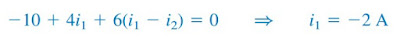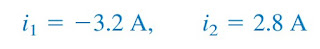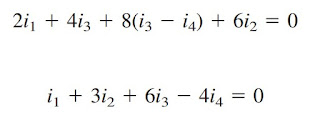omG8HI9LSKFW6lhCj8prTs0Z6lUhdOT9Jhi1Sf4m

# Supermesh Electric Circuit Analysis - Problem Solving

Have you learned about mesh analysis completely? Is it easy or hard to understand? If you think the previous post about mesh current method or mesh analysis is quite hard, try to read it one more time.

Like we stated in that post, we have limited the use of the current source in a circuit if we want to use mesh analysis. Applying mesh analysis to circuits containing current sources (dependent or independent) may appear complicated.

Is mesh analysis can't analyze an electric circuit containing current sources?

Of course not, we only limited the current source to make you the basic principle with the easiest approach.

If the current source is found just in a single branch then it is easy to solve, we can use the mesh analysis directly.

We will need another version of mesh analysis if we found the current source in a branch where two meshes intersect.

Now we will use current sources in a circuit and analyze it with mesh analysis or we may call it Supermesh Analysis.

Make sure to read what is dc circuit first.

## Mesh Analysis with Current Sources

Even we need to modify the mesh current method analysis a little bit, let me be honest with you the good thing about it.

The current sources will reduce the number of equations, makes it easier to solve. Let us take a look at two possibilities.

CASE 1 -  When a current source exists only in one mesh: Consider in Figure.(1) for example,Fig.1 Supermesh analysis electric circuit
we set i2 = -5 A and write equation for the other mesh in the usual ways; that is,(1)
CASE 2 - When a current source exists between two meshes: Consider the circuit in Figure.(2a), for example.Figure 2. Supermesh with intersect
We create a supermesh by excluding the current source and any elements connected in series with it, as shown in Figure.(2b). Thus,
A supermesh results when two meshes have a (dependent or independent) current source in common.
As shown in Figure.(2b), we create a supermesh as the periphery of the two meshes and treat it differently. (If a circuit has two or more supermeshes that intersect, they should be combined to form a larger supermesh). Why treat supermesh differently? Because mesh analysis applies KVL - which requires that we know the voltage across each branch - and we do not know the voltage across the current source in advance. However, a supermesh must satisfy KVL like any other mesh. Therefore, applying KVL to the supermesh in Figure.(2b) gives(2)
We apply KCL to a node in the branch where the two meshes intersect. Applying KCL to node 0 in Figure.(2a) gives(3)
Solving Equations.(2) and (3), we get(4)
Note the following properties of a supermesh :
1. The current source in the supermesh provides the constraint equation necessary to solve for the mesh currents.
2. A supermesh has no current of its own.
3. A supermesh requires the application of both KVL and KCL.

## Procedure of Supermesh Analysis

This is the summary of supermesh analysis' step-by-step
1. Make sure the circuit is planar.
2. Redraw the circuit if we can simplify it.
3. Make meshes in every loop you can find and assign the labels. It is easier to draw the meshes in the clockwise direction (it is up to you, though).
4. Form a supermesh if you find a current source between two meshes.
5. Use KVL and maybe some KCL to the supermesh branch. One KCL is needed for each supermesh branch.
6. Solve all the math equations.

## Supermesh Analysis Examples

For better understanding, let us review the example below :
1. For the circuit in Figure.(3), find i1 to i4 using mesh analysis.Figure 3
Solution :
Note that meshes 1 and 2 form a supermesh since they have an independent current source in common. Also, meshes 2 and 3 form another supermesh because they have dependent current source in common. The two supermeshes intersect and form a larger supermesh as shown. Applying KVL to the larger supermesh,(1.1)
For the independent current source, we apply KCL to node P :(1.2)
For the dependent current source, we apply KCL to node Q :
But Io = - i4 , thus,(1.3)
Applying KVL in mesh 4,(1.4)
From (1.1) to (1.4),

Have you understood what is supermesh analysis? Don't forget to share and subscribe! Happy learning!
Reference:  Fundamentals of electric circuits by Charles K. Alexander and Matthew N. O. Sadiku

Untuk Bahasa Indonesia baca Analisis Supermesh.
Related Posts
SHARE